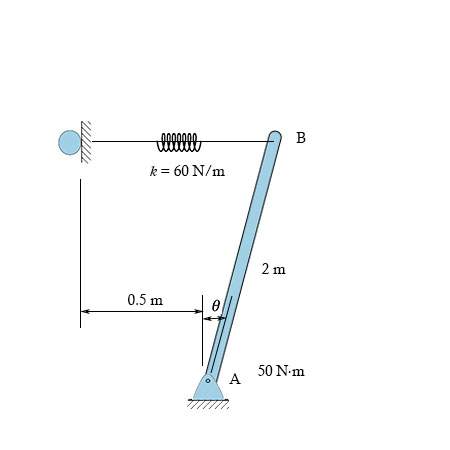# The 10.2 kg uniform rod is pinned at end A. If it is also subjected to a couple moment of 50...

## Question:

The 10.2 kg uniform rod is pinned at end A. If it is also subjected to a couple moment of 50 N {eq}\cdot {/eq} m. The spring is unstretched when {eq}\theta {/eq} = 0, and has a stiffness of {eq}k {/eq} = 60 N/m. Determine the smallest angle {eq}\theta {/eq} for equilibrium.## Equilibrium of forces.

The equilibrium of forces is achieved by resolving the forces in x and y directions, the forces are resolved according to the total load acting on that point.

Given data

Mass of the rod, {eq}m = 10.2\;{\rm{kg}} {/eq}

The couple moment, {eq}M = 50\;{\rm{N}} \cdot {\rm{m}} {/eq}

Spring stiffness {eq}k =...

Become a Study.com member to unlock this answer! Create your account